# Measurement of the output impedance of any quadrupole

#### ♦ Example 1: the headphone output of a laptop computer ( Sony VAIO )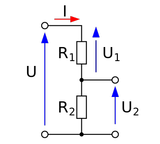We consider the output of the quadrupole as a generator. There are two very simple solutions to quantify this data:

• the first consists in replacing the load (R 2 ) by a resistor whose value is known, and in measuring the voltage at its terminals (U 2 ).
The equation is then solved simply.

• the second uses the principle of half-voltage: a variable potentiometer is used instead of resistor R 2 , and its value is varied until a voltage is obtained that is half that of the generator U 2 = U / 2

The two resistors R 1 and R 2 are then of equal values. All that remains is to measure the resulting value of the potentiometer using an ohmmeter.

Materials required:

✔ oscilloscope

✔ multimeter

First method :

This method is the easiest to implement if one knows the magnitude of the ohmic value to be sought, otherwise it will be necessary to experiment with different values ​​until a satisfactory result is obtained. To simplify the calculations, it will be considered that the divider bridge is made up of pure resistors.

According to Thévenin's theorem and with the help of Ohm's law , the generator voltage being known, it suffices to give a known value to resistance R 2 to easily deduce the value of resistance R 1 . During this manipulation, I use for R 2 a value of 8.2 Ω which I simply have in my drawers, but other values ​​may be equally suitable, it all depends on the size of the value to be sought.

The generator supplies a voltage U via its internal resistance R 1 which can be assimilated to the output impedance of the amplifier. The measurement resistor R 2 allows us to visualize the output voltage U 2 on an oscilloscope.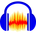The MP3 signal recorded with Audacity software provides a sinusoidal signal of frequency 1 KHz and 1 VC / C - 0 dB. No-load, that is to say without the measurement resistor R 2 , the voltage present at the output is therefore equal to that of the generator, ie 1 VC / C.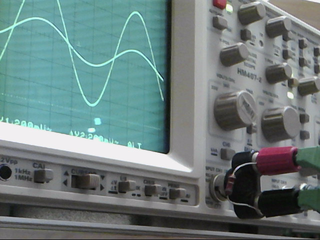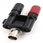The measurement resistor is connected in parallel to an oscilloscope input via, for example, a coaxial-BNC adapter ( see the photo opposite ). A single shielded cable with a stereo jack plug is connected to the headphone output of the PC, the other stripped end is connected to the input (CH1) of the oscilloscope. Optionally it is possible to connect the second channel to the CH2 input for comparison. Under these conditions, the two voltages U 2 and U are displayed simultaneously.

When the resistor R 2 is connected , the voltage U 2 is then reduced due to the series connection of the two resistors, here we obtain a voltage divider bridge .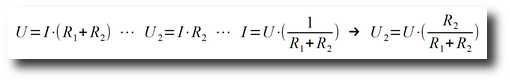After having carried out the assembly and carried out the measurement, let's proceed to quick calculations: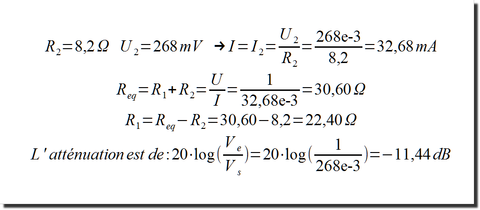The output impedance of the laptop PC amplifier (headphone output) is therefore approximately 22 Ω. On the screenshot below from my calculator's solver the parameters are:

• U corresponds to the voltage U of the generator

• V corresponds to the output voltage U 2

• X corresponds to the output resistance R 1

• R corresponds to the measurement resistance R 2

We find here the equivalence of our starting formula: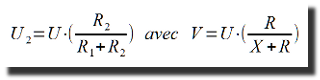#### ♦ Example 2: the 50 Ω output of a low frequency generator ( GBF )

For this manipulation, I used a shielded cable with two BNC plugs, a BNC tee and the coaxial-BNC adapter mentioned above.
The generator set to the frequency of 1 KHz for a level of 1 V C / C returned the following values ​​to me: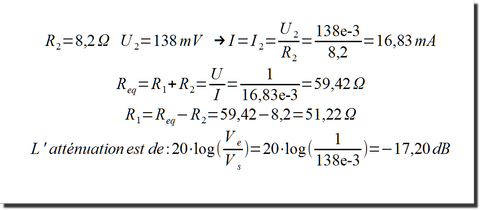The output impedance of the GBF generator is therefore approximately 51 Ω.I tried the experiment with a 100 Ω and this time the value obtained is 51.97 Ω ...
The result is very consistent with the characteristics of the device being measured, and this demonstrates without any ambiguity the reliability of the test which remains very simple!

#### ♦ Example 3: the headphone output of a smartphone ( OnePlus 5 )

The test carried out still under the same conditions as in example 1 returned the following values: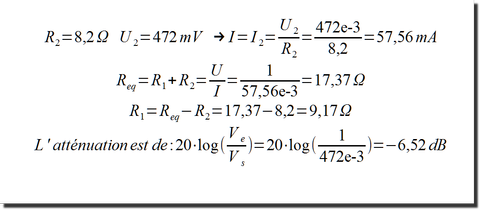The output impedance of the smartphone amplifier ( headphone output ) is therefore approximately 9 Ω.

Second method :

To use this method, it is necessary to have a potentiometer or variable resistor with a value greater than the impedance to be measured.
In this case, the potentiometer replaces the measurement resistor R 2 , which must be adjusted so as to obtain on the oscilloscope screen a voltage of half that delivered by the generator.
This condition having been fulfilled, all that remains is to disconnect the variable resistor to measure its value using an ohmmeter.
The method can be applied to any quadrupole whatever it is.

### Licence Creative Commons

L'ensemble de ce site hormis une notification spécifique est mis
à  disposition selon les termes de la
Attribution - Pas d'Utilisation Commerciale - Partage dans les Mêmes Conditions
3.0 France.### Mes fournisseurs favoris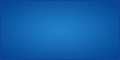Vérifiez la disponibilité de votre nom de domaine :
 fr com mobi eu net org info name co biz cc tv ws